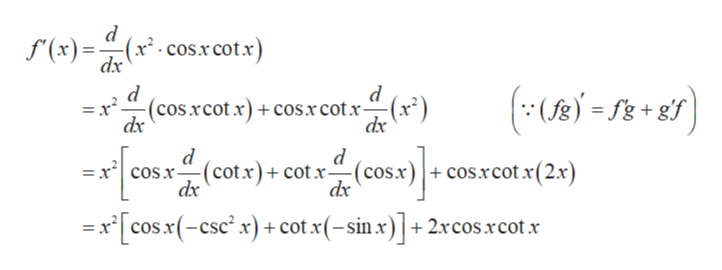# differentiate the following function f(x)=x2Cos(x)Cot(x)

Question
15 views

differentiate the following function

f(x)=x2Cos(x)Cot(x)

check_circle

Step 1

The given function is,

Step 2

Use product rule to differentiate the function...help_outlineImage Transcriptionclosed -(x2- cos.x cotx) dx (x)= d (cos xcot x) +cos.xcotx- d ()f d = x' cos.r-(cotx)+ cotx--(cos.x)+cos.xcot x(2.x dx =x cosx(-csc x)cot x(-sin x)+2xcos.rcot.x fullscreen

### Want to see the full answer?

See Solution

#### Want to see this answer and more?

Solutions are written by subject experts who are available 24/7. Questions are typically answered within 1 hour.*

See Solution
*Response times may vary by subject and question.
Tagged in

### Derivative